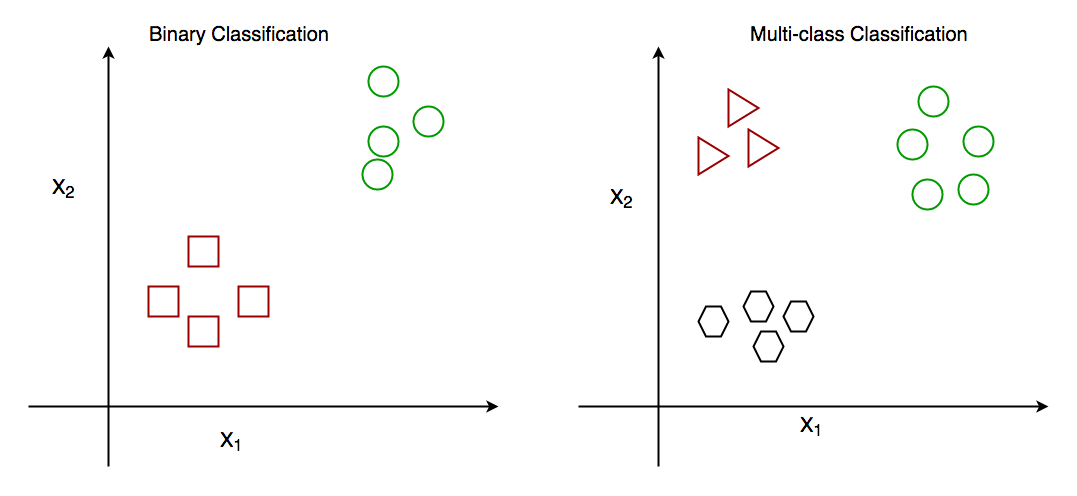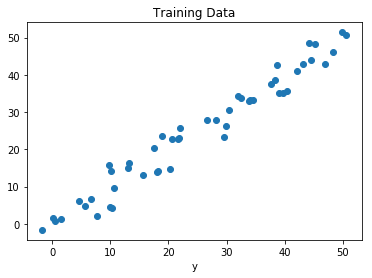# Classification vs Regression

Classification and Regression are two major prediction problems which are usually dealt with Data mining and machine learning.

Classification is the process of finding or discovering a model or function which helps in separating the data into multiple categorical classes i.e. discrete values. In classification, data is categorized under different labels according to some parameters given in input and then the labels are predicted for the data.
The derived mapping function could be demonstrated in the form of “IF-THEN” rules. The classification process deal with the problems where the data can be divided into binary or multiple discrete labels.

Let’s take an example, suppose we want to predict the possibility of the wining of match by Team A on the basis of some parameters recorded earlier. Then there would be two labels Yes and No.Fig : Binary Classification and Multiclass Classification

Regression is the process of finding a model or function for distinguishing the data into continuous real values instead of using classes or discrete values. It can also identify the distribution movement depending on the historical data. Because a regression predictive model predicts a quantity, therefore, the skill of the model must be reported as an error in those predictions
Let’s take a similar example in regression also, where we are finding the possibility of rain in some particular regions with the help of some parameters recorded earlier. Then there is a probability associated with the rain.Fig : Regression of Day vs Rainfall (in mm)

Comparison between Clasification and Regression:

PARAMENTER CLASSIFICATION REGRESSION
Basic Mapping Function is used for mapping of values to predefined classes. Mapping Function is used for mapping of values to continuous output.
Involves prediction of Discrete values Continuous values
Nature of the predicted data Unordered Ordered
Method of calculation by measuring accuracy by measurement of root mean square error
Example Algorithms Decision tree, logistic regression, etc. Regression tree (Random forest), Linear regression, etc.

# Special 95% discount

## 2000+ Applied Machine Learning & Data Science Recipes

### Portfolio Projects for Aspiring Data Scientists: Tabular Text & Image Data Analytics as well as Time Series Forecasting in Python & R## Two Machine Learning Fields

There are two sides to machine learning:

• Practical Machine Learning:This is about querying databases, cleaning data, writing scripts to transform data and gluing algorithm and libraries together and writing custom code to squeeze reliable answers from data to satisfy difficult and ill defined questions. It’s the mess of reality.
• Theoretical Machine Learning: This is about math and abstraction and idealized scenarios and limits and beauty and informing what is possible. It is a whole lot neater and cleaner and removed from the mess of reality.

Data Science Resources: Data Science Recipes and Applied Machine Learning Recipes

Introduction to Applied Machine Learning & Data Science for Beginners, Business Analysts, Students, Researchers and Freelancers with Python & R Codes @ Western Australian Center for Applied Machine Learning & Data Science (WACAMLDS) !!!

Latest end-to-end Learn by Coding Recipes in Project-Based Learning:

Applied Statistics with R for Beginners and Business Professionals

Data Science and Machine Learning Projects in Python: Tabular Data Analytics

Data Science and Machine Learning Projects in R: Tabular Data Analytics

Python Machine Learning & Data Science Recipes: Learn by Coding

R Machine Learning & Data Science Recipes: Learn by Coding

Comparing Different Machine Learning Algorithms in Python for Classification (FREE)

`Disclaimer: The information and code presented within this recipe/tutorial is only for educational and coaching purposes for beginners and developers. Anyone can practice and apply the recipe/tutorial presented here, but the reader is taking full responsibility for his/her actions. The author (content curator) of this recipe (code / program) has made every effort to ensure the accuracy of the information was correct at time of publication. The author (content curator) does not assume and hereby disclaims any liability to any party for any loss, damage, or disruption caused by errors or omissions, whether such errors or omissions result from accident, negligence, or any other cause. The information presented here could also be found in public knowledge domains.  `